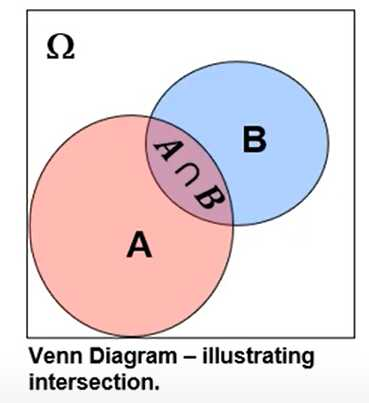# [GeostatsGuy Lectures] Bayesian Probability

## Derivation

Product Rule:

\begin{aligned}
&P(B \cap A)=P(A \mid B) P(B) \\
&P(A \cap B)=P(B \mid A) P(A)
\end{aligned}

It follows that:

P(B \cap A)=P(A \cap B)

Therefore we combine two product rules, substitute:

P(A \mid B) P(B)=P(B \mid A) P(A)

We get Bayes' Theorem!

Bayesian Statistical Approaches:
- probabilities based on:
- state of knowledge
- degree of belief in an event
- utilize an assessment prior to data collection
- updated as new information is available
- solve probability problems that we cannot use simple frequencies

• Bayesian credibility intervals provide a more intuitive measure of uncertainty than Frequentist confidence intervals, more later...

Bayes' Theorem:
Make an easy adjustment and we get the popular form.

P^{\top}(A, B)=\frac{P(B \mid A) P(A)}{P(B)}

Observations:

1. We can get P(A \mid B) from P(B \mid A), as you will see this often comes in handy.
2. Each term is known as:

Posterior =\frac{\text { Likelihood } \mathrm{x} \text { Prior }}{\text { Evidence }}

1. Prior should have no information from likelihood.
2. Evidence term is usually just a standardization to ensure closure.## Summary## Bayes Theorem: Alternative form,

symmetry:

P(A \mid B)=\frac{P(B \mid A) P(A)}{P(B)} \quad P(B \mid A)=\frac{P(A \mid B) P(B)}{P(A)}

Alternative form to calculate evidence term:

Given:
$P(A)=\underbrace{P(A \mid B) P(B)}{P(A \text { and } B)}+\underbrace{P\left(A \mid B^{c}\right) P\left(B^{c}\right)}{P\left(A \text { and } B^{c}\right)}$

P(B \mid A)=\frac{P(A \mid B) P(B)}{P(A)}=\frac{P(A \mid B) P(B)}{P(A \mid B) P(B)+P\left(A \mid B^{c}\right) P\left(B^{c}\right)}

## Applications of Beyes' Theorem